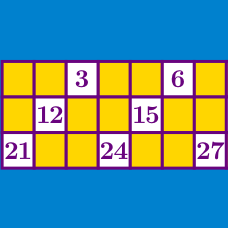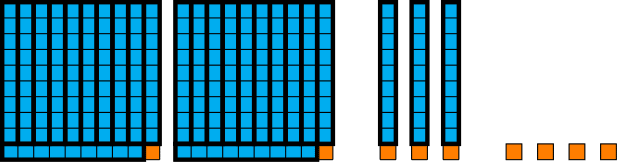Number Theory

Divisibility Tests Warmup

Is 12345 divisible by 5?

Note: 12345 is divisible by 5 if it can be divided into groups of 5 with nothing left over.Is 234 divisible By 9?

A number that consists entirely of 1s (like 1, 11, 111, etc.) is called a repunit. How many digits long is the smallest repunit that is divisible by 9?

For how many different values of $A$ is $27A4$ (a four digit number where the third digit is $A$) divisible by 6?

For how many different values of $A$ is the three digit number $3A6$ even?

Note: $A$ can be any of the 10 digits from 0 to 9.

×